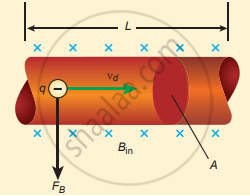Tamil Nadu Board of Secondary EducationHSC Science Class 12th

# Derive the expression for the force on a current-carrying conductor in a magnetic field. - Physics

Numerical

Derive the expression for the force on a current-carrying conductor in a magnetic field.

#### Solution

1. The force experienced is equal to the sum of Lorentz forces on the individual charge carriers in the wire.
2. Consider a small length of wire ‘dl’, area – A, Current – I.
3. The free electrons drift opposite to the direction of the current.
4. The relation between I & Vd is given by
I = neAV5. In magnetic field, the force experienced is given by
vec"F" = - "e" (vec"v"_"d" xx vec"B")
n → number of free electrons per unit volume.
n = "N"/"V"
V = A dl
6. Lorentz force = n A dl × – "e"(vec"v"_"d" xx vec"B")
dF = -enA dl (vec"v"_"d" xx vec"B")
7. Current element, "I"vec"dl" = - "enA"vec"v"_"d"
therefore "d"vec"F" = ("l" vec"dl" = vec"B")
vec"F" = ("l"  vec"l" xx vec"B")
F = BIl sin θ
Case (i):
If the conductor is along the magnetic field; θ = 0° ; F = 0.
Case (ii):
If the conductor is perpendicular to the magnetic field; θ = 90°; F = BIl.
Concept: Magnetic Effects of Current
Is there an error in this question or solution?

#### APPEARS IN

Tamil Nadu Board Samacheer Kalvi Class 12th Physics Volume 1 and 2 Answers Guide
Chapter 3 Magnetism and magnetic effects of electric current
Evaluation | Q III. 16. | Page 192
Share